# RS Aggarwal Solutions For Class 7 Maths Exercise 2B Chapter 2 Fractions

The pdf of RS Aggarwal Solutions for the Exercise 2B of Class 7 Maths Chapter 2, Fractions are available here. To cover all the syllabus in Maths, the RS Aggarwal is an essential material as it offers a wide range of questions that test the students’ understanding of concepts. Our expert personnel have solved the problems step by step with neat explanations. Students who want to score good marks in Maths, then refer RS Aggarwal Solutions for Class 7.

## Download the PDF of RS Aggarwal Solutions For Class 7 Maths Chapter 2 Fractions – Exercise 2B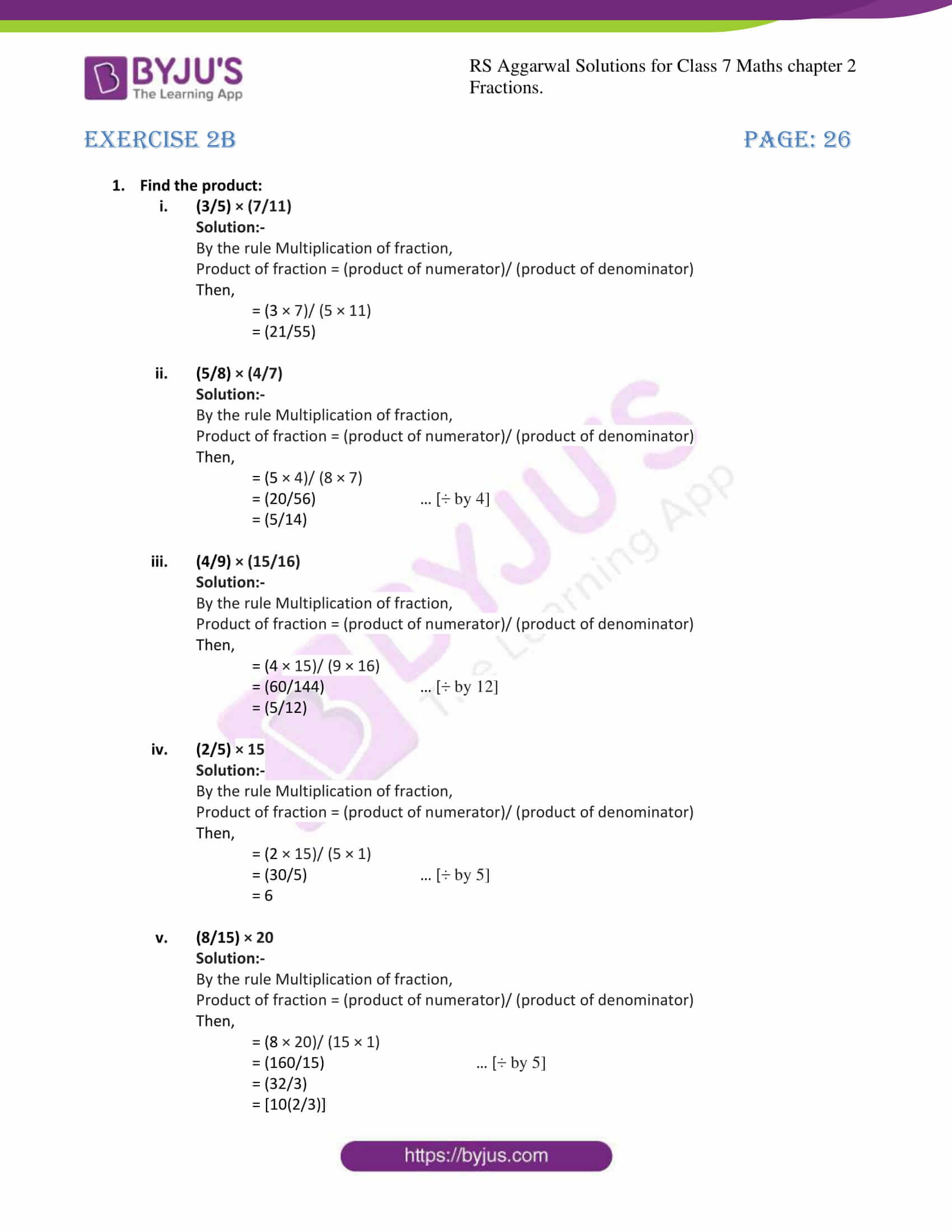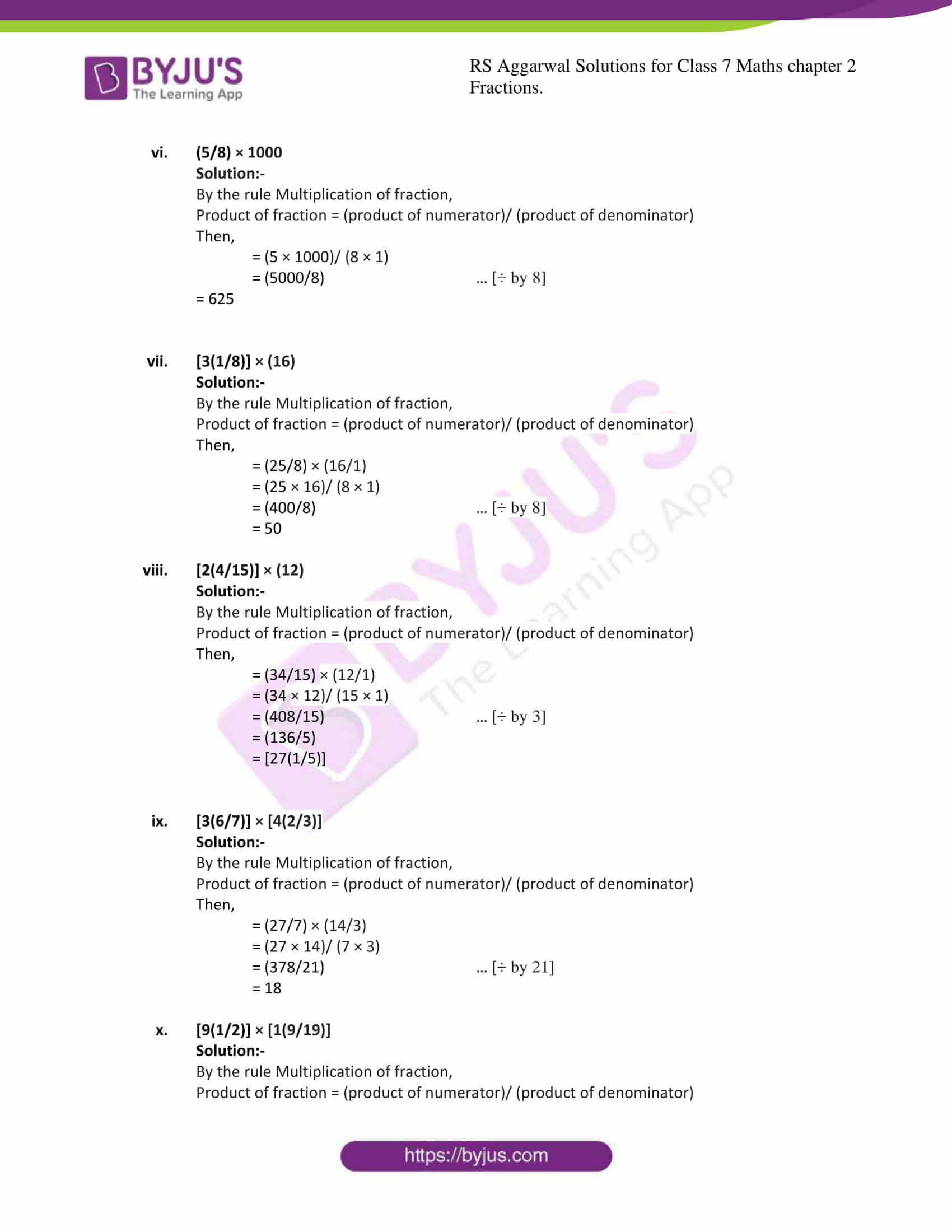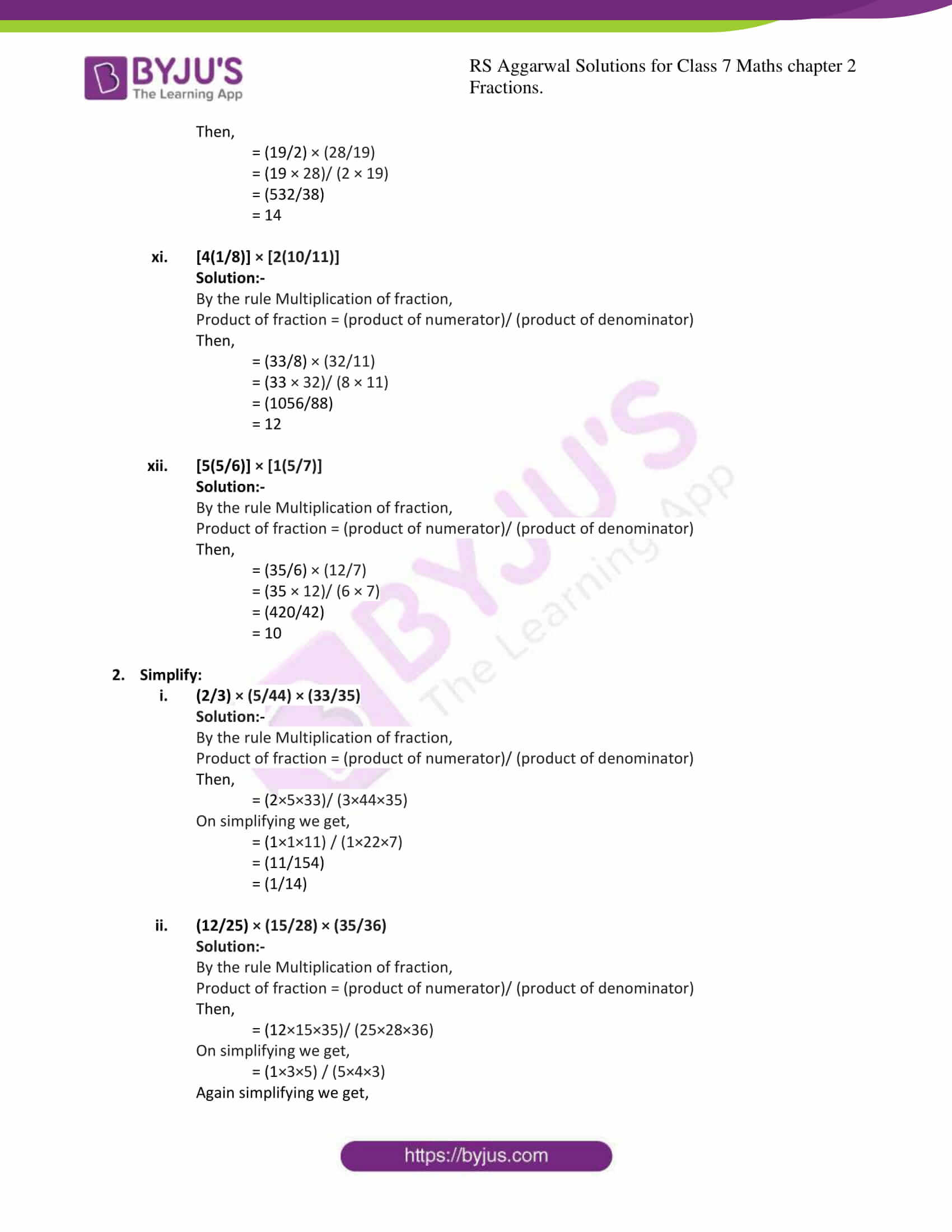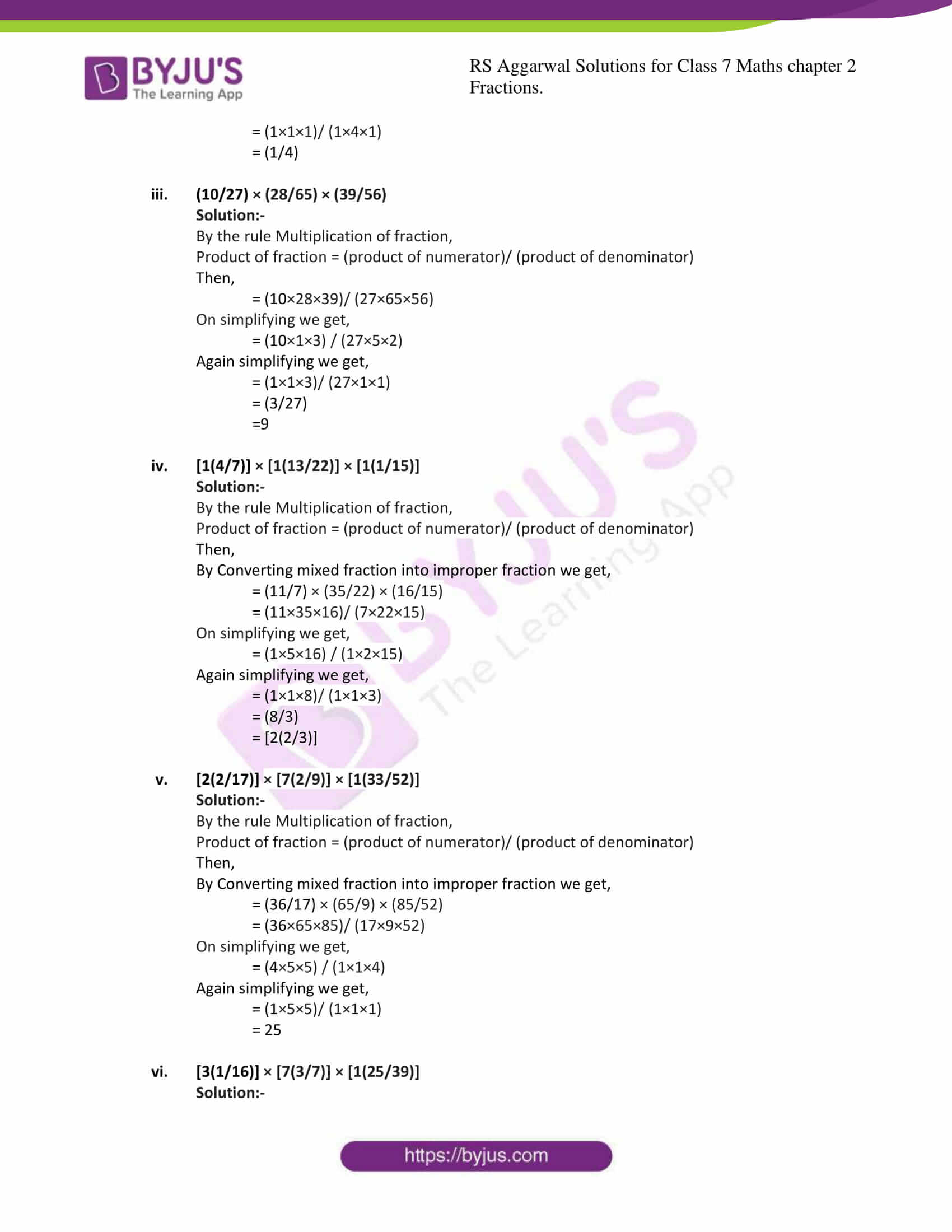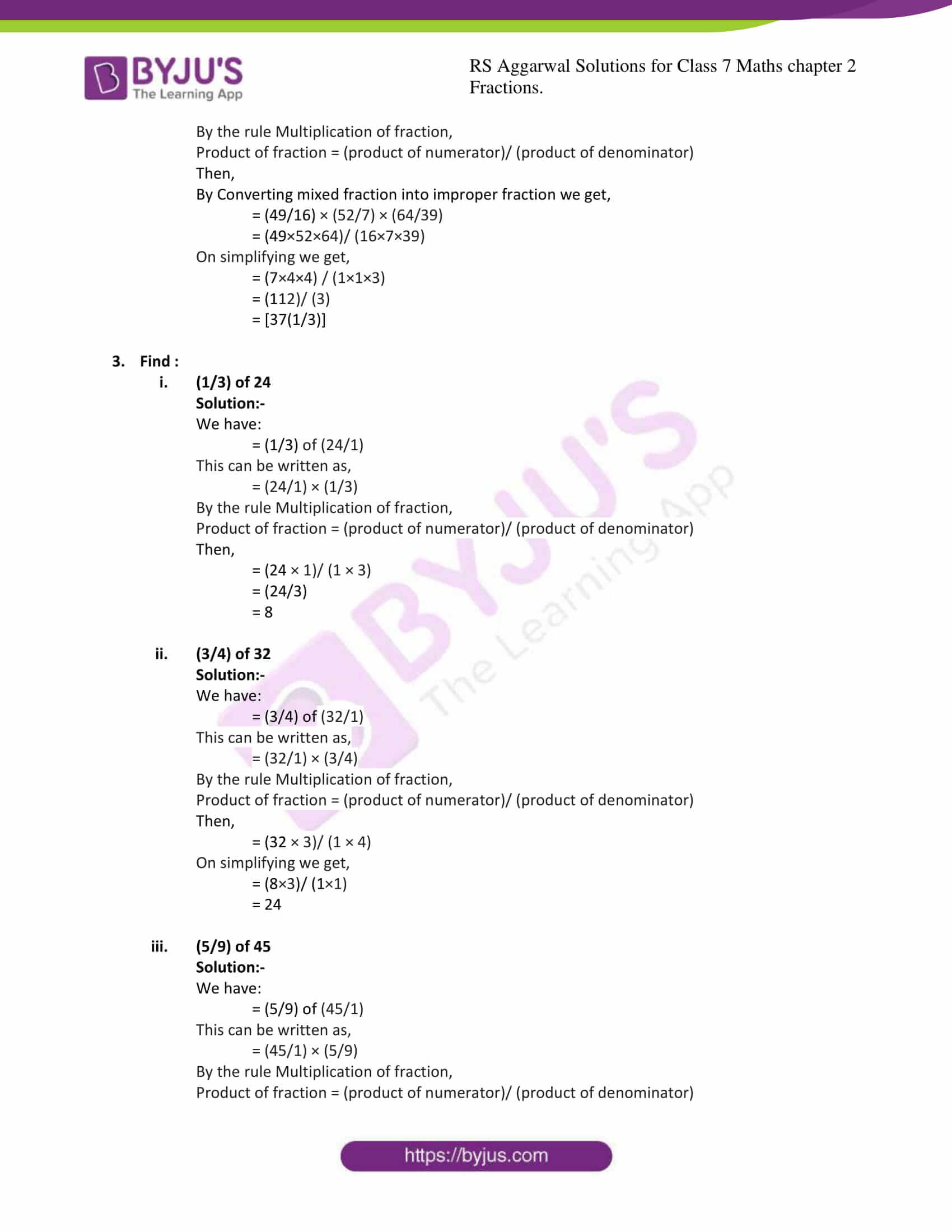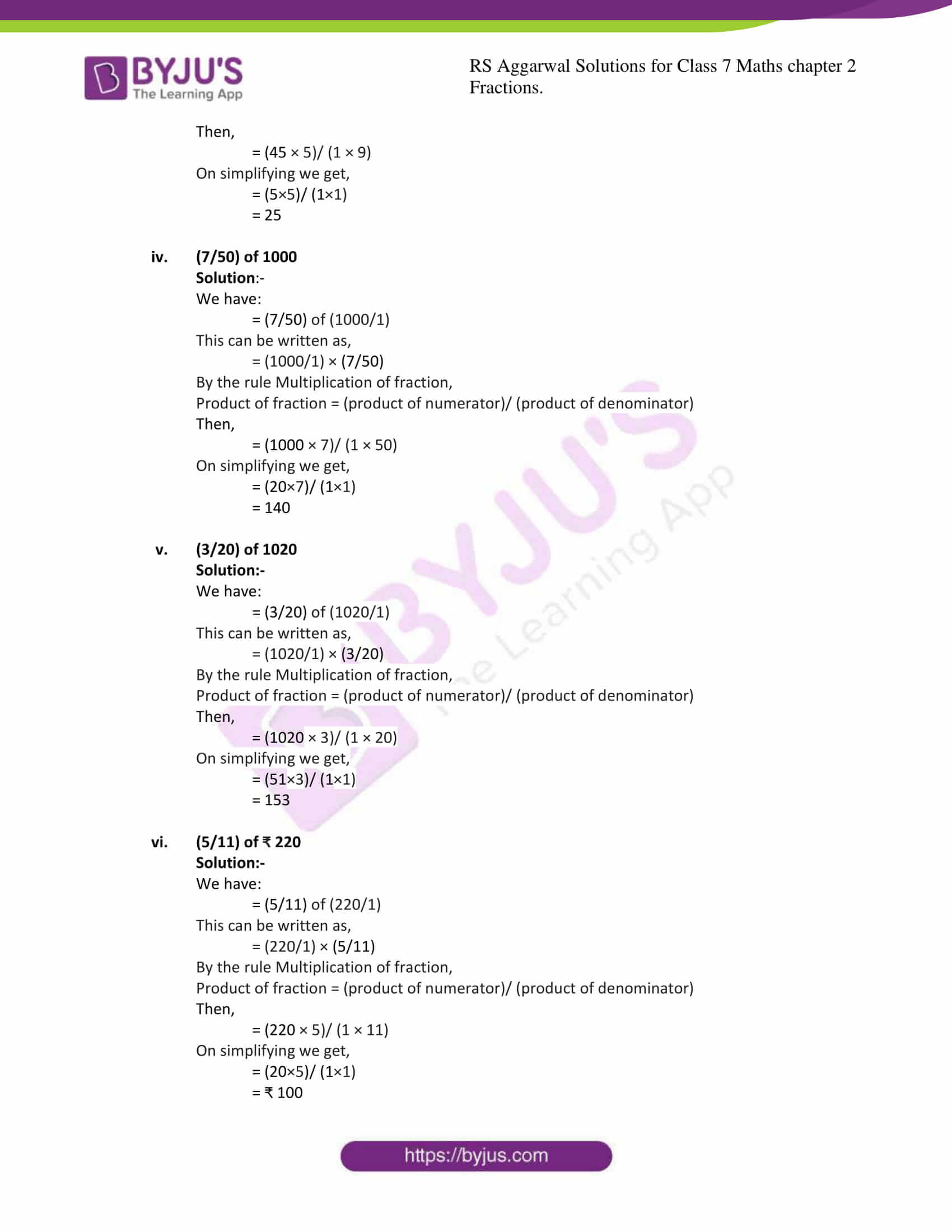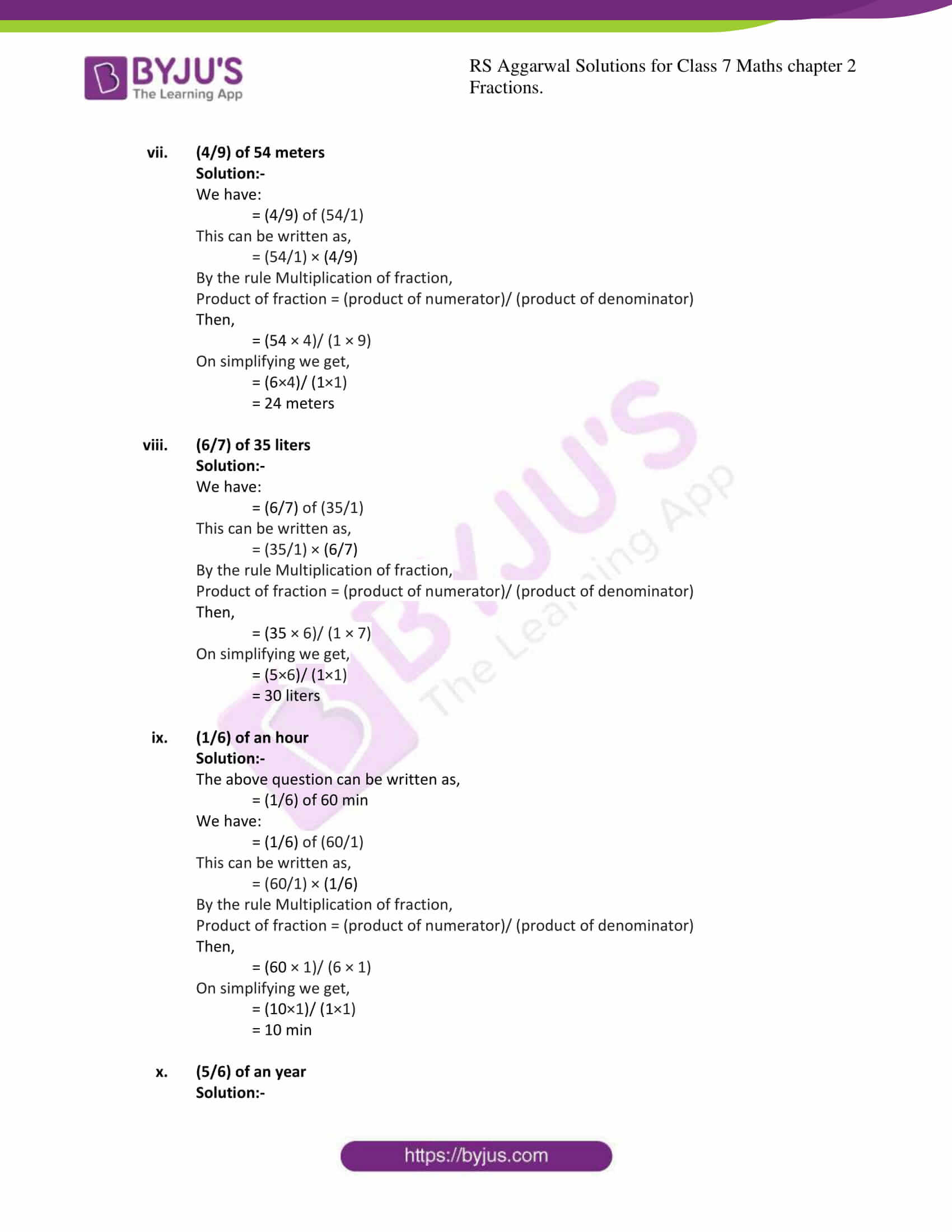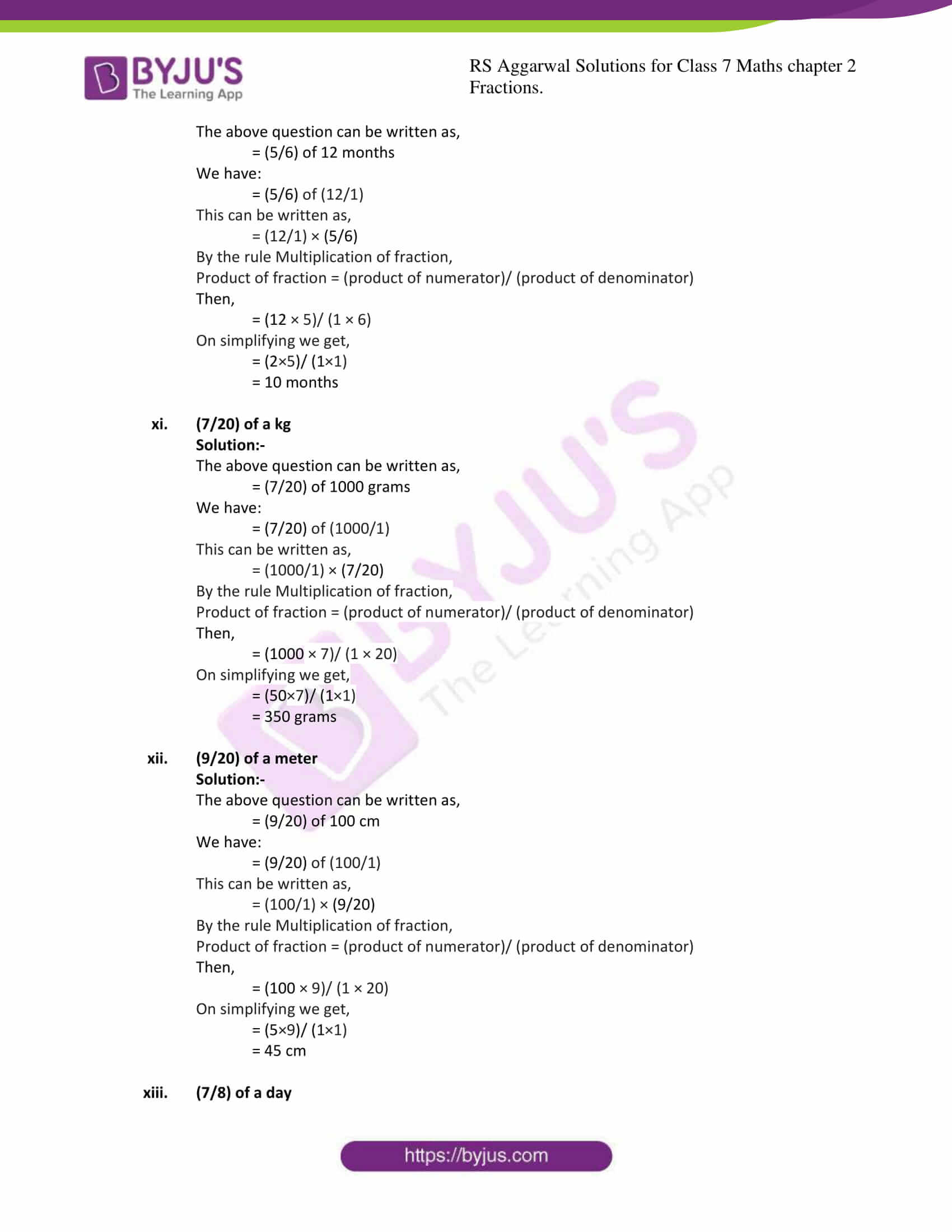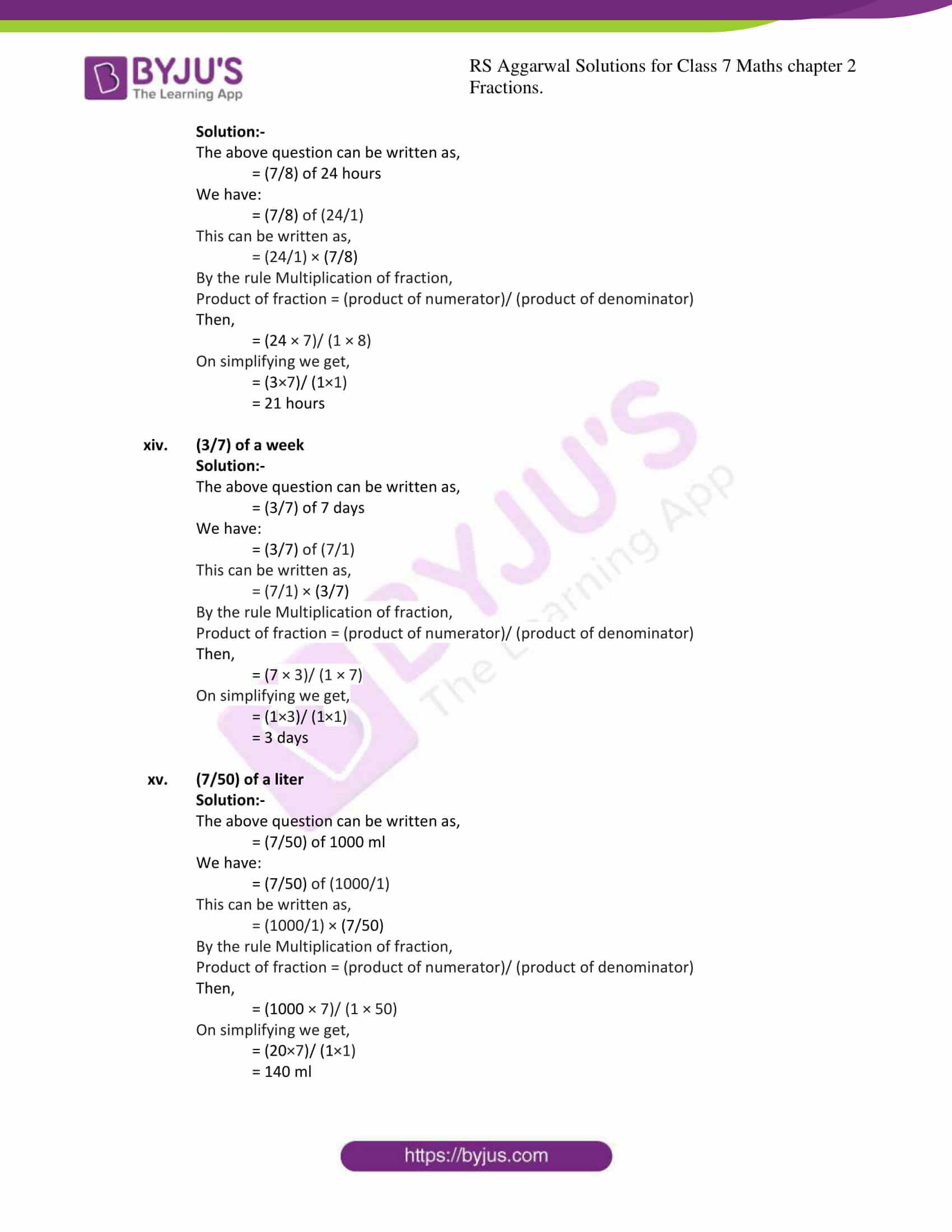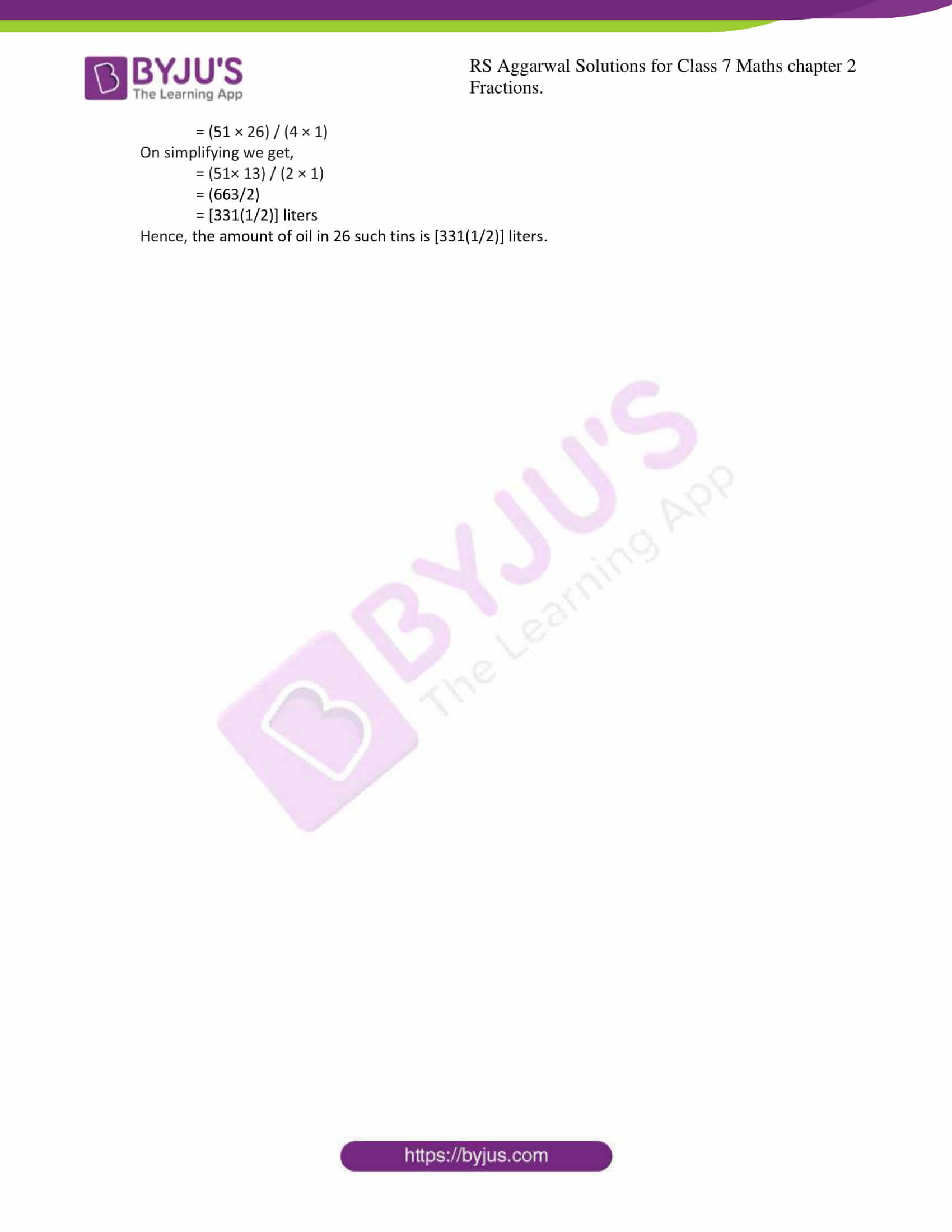### Access answers to Maths RS Aggarwal Solutions for Class 7 Chapter 2 – Fractions Exercise 2B

1. Find the product:
(i) (3/5) × (7/11)

Solution:-

By the rule Multiplication of fraction,

Product of fraction = (product of numerator)/ (product of denominator)

Then,

= (3 × 7)/ (5 × 11)

= (21/55)

(ii) (5/8) × (4/7)

Solution:-

By the rule Multiplication of fraction,

Product of fraction = (product of numerator)/ (product of denominator)

Then,

= (5 × 4)/ (8 × 7)

= (20/56) … [÷ by 4]

= (5/14)

(iii) (4/9) × (15/16)

Solution:-

By the rule Multiplication of fraction,

Product of fraction = (product of numerator)/ (product of denominator)

Then,

= (4 × 15)/ (9 × 16)

= (60/144) … [ by 12]

= (5/12)

(iv) (2/5) × 15

Solution:-

By the rule Multiplication of fraction,

Product of fraction = (product of numerator)/ (product of denominator)

Then,

= (2 × 15)/ (5 × 1)

= (30/5) … [÷ by 5]

= 6

(v) (8/15) × 20

Solution:-

By the rule Multiplication of fraction,

Product of fraction = (product of numerator)/ (product of denominator)

Then,

= (8 × 20)/ (15 × 1)

= (160/15) … [÷ by 5]

= (32/3)

= [10(2/3)]

(vi) (5/8) × 1000

Solution:-

By the rule Multiplication of fraction,

Product of fraction = (product of numerator)/ (product of denominator)

Then,

= (5 × 1000)/ (8 × 1)

= (5000/8) … [÷ by 8]

= 625

(vii)[3(1/8)] × (16)

Solution:-

By the rule Multiplication of fraction,

Product of fraction = (product of numerator)/ (product of denominator)

Then,

= (25/8) × (16/1)

= (25 × 16)/ (8 × 1)

= (400/8) … [÷ by 8]

= 50

(viii)[2(4/15)] × (12)

Solution:-

By the rule Multiplication of fraction,

Product of fraction = (product of numerator)/ (product of denominator)

Then,

= (34/15) × (12/1)

= (34 × 12)/ (15 × 1)

= (408/15) … [÷ by 3]

= (136/5)

= [27(1/5)]

(ix)[3(6/7)] × [4(2/3)]

Solution:-

By the rule Multiplication of fraction,

Product of fraction = (product of numerator)/ (product of denominator)

Then,

= (27/7) × (14/3)

= (27 × 14)/ (7 × 3)

= (378/21) … [÷ by 21]

= 18

(x) [9(1/2)] × [1(9/19)]

Solution:-

By the rule Multiplication of fraction,

Product of fraction = (product of numerator)/ (product of denominator)

Then,

= (19/2) × (28/19)

= (19 × 28)/ (2 × 19)

= (532/38)

= 14

(xi) [4(1/8)] × [2(10/11)]

Solution:-

By the rule Multiplication of fraction,

Product of fraction = (product of numerator)/ (product of denominator)

Then,

= (33/8) × (32/11)

= (33 × 32)/ (8 × 11)

= (1056/88)

= 12

(xii) [5(5/6)] × [1(5/7)]

Solution:-

By the rule Multiplication of fraction,

Product of fraction = (product of numerator)/ (product of denominator)

Then,

= (35/6) × (12/7)

= (35 × 12)/ (6 × 7)

= (420/42)

= 10

2. Simplify:
(i) (2/3) × (5/44) × (33/35)

Solution:-

By the rule Multiplication of fraction,

Product of fraction = (product of numerator)/ (product of denominator)

Then,

= (2×5×33)/ (3×44×35)

On simplifying we get,

= (1×1×11) / (1×22×7)

= (11/154)

= (1/14)

(ii) (12/25) × (15/28) × (35/36)

Solution:-

By the rule Multiplication of fraction,

Product of fraction = (product of numerator)/ (product of denominator)

Then,

= (12×15×35)/ (25×28×36)

On simplifying we get,

= (1×3×5) / (5×4×3)

Again simplifying we get,

= (1×1×1)/ (1×4×1)

= (1/4)

(iii) (10/27) × (28/65) × (39/56)

Solution:-

By the rule Multiplication of fraction,

Product of fraction = (product of numerator)/ (product of denominator)

Then,

= (10×28×39)/ (27×65×56)

On simplifying we get,

= (10×1×3) / (27×5×2)

Again simplifying we get,

= (1×1×3)/ (27×1×1)

= (3/27)

=9

(iv) [1(4/7)] × [1(13/22)] × [1(1/15)]

Solution:-

By the rule Multiplication of fraction,

Product of fraction = (product of numerator)/ (product of denominator)

Then,

By Converting mixed fraction into improper fraction we get,

= (11/7) × (35/22) × (16/15)

= (11×35×16)/ (7×22×15)

On simplifying we get,

= (1×5×16) / (1×2×15)

Again simplifying we get,

= (1×1×8)/ (1×1×3)

= (8/3)

= [2(2/3)]

(v) [2(2/17)] × [7(2/9)] × [1(33/52)]

Solution:-

By the rule Multiplication of fraction,

Product of fraction = (product of numerator)/ (product of denominator)

Then,

By Converting mixed fraction into improper fraction we get,

= (36/17) × (65/9) × (85/52)

= (36×65×85)/ (17×9×52)

On simplifying we get,

= (4×5×5) / (1×1×4)

Again simplifying we get,

= (1×5×5)/ (1×1×1)

= 25

(vi) [3(1/16)] × [7(3/7)] × [1(25/39)]

Solution:-

By the rule Multiplication of fraction,

Product of fraction = (product of numerator)/ (product of denominator)

Then,

By Converting mixed fraction into improper fraction we get,

= (49/16) × (52/7) × (64/39)

= (49×52×64)/ (16×7×39)

On simplifying we get,

= (7×4×4) / (1×1×3)

= (112)/ (3)

= [37(1/3)]

3. Find :
(i) (1/3) of 24

Solution:-

We have:

= (1/3) of (24/1)

This can be written as,

= (24/1) × (1/3)

By the rule Multiplication of fraction,

Product of fraction = (product of numerator)/ (product of denominator)

Then,

= (24 × 1)/ (1 × 3)

= (24/3)

= 8

(ii) (3/4) of 32

Solution:-

We have:

= (3/4) of (32/1)

This can be written as,

= (32/1) × (3/4)

By the rule Multiplication of fraction,

Product of fraction = (product of numerator)/ (product of denominator)

Then,

= (32 × 3)/ (1 × 4)

On simplifying we get,

= (8×3)/ (1×1)

= 24

(iii) (5/9) of 45

Solution:-

We have:

= (5/9) of (45/1)

This can be written as,

= (45/1) × (5/9)

By the rule Multiplication of fraction,

Product of fraction = (product of numerator)/ (product of denominator)

Then,

= (45 × 5)/ (1 × 9)

On simplifying we get,

= (5×5)/ (1×1)

= 25

(iv) (7/50) of 1000

Solution:-

We have:

= (7/50) of (1000/1)

This can be written as,

= (1000/1) × (7/50)

By the rule Multiplication of fraction,

Product of fraction = (product of numerator)/ (product of denominator)

Then,

= (1000 × 7)/ (1 × 50)

On simplifying we get,

= (20×7)/ (1×1)

= 140

(v) (3/20) of 1020

Solution:-

We have:

= (3/20) of (1020/1)

This can be written as,

= (1020/1) × (3/20)

By the rule Multiplication of fraction,

Product of fraction = (product of numerator)/ (product of denominator)

Then,

= (1020 × 3)/ (1 × 20)

On simplifying we get,

= (51×3)/ (1×1)

= 153

(vi) (5/11) of ₹ 220

Solution:-

We have:

= (5/11) of (220/1)

This can be written as,

= (220/1) × (5/11)

By the rule Multiplication of fraction,

Product of fraction = (product of numerator)/ (product of denominator)

Then,

= (220 × 5)/ (1 × 11)

On simplifying we get,

= (20×5)/ (1×1)

= ₹ 100

(vii) (4/9) of 54 meters

Solution:-

We have:

= (4/9) of (54/1)

This can be written as,

= (54/1) × (4/9)

By the rule Multiplication of fraction,

Product of fraction = (product of numerator)/ (product of denominator)

Then,

= (54 × 4)/ (1 × 9)

On simplifying we get,

= (6×4)/ (1×1)

= 24 meters

(viii) (6/7) of 35 liters

Solution:-

We have:

= (6/7) of (35/1)

This can be written as,

= (35/1) × (6/7)

By the rule Multiplication of fraction,

Product of fraction = (product of numerator)/ (product of denominator)

Then,

= (35 × 6)/ (1 × 7)

On simplifying we get,

= (5×6)/ (1×1)

= 30 liters

(ix) (1/6) of an hour

Solution:-

The above question can be written as,

= (1/6) of 60 min

We have:

= (1/6) of (60/1)

This can be written as,

= (60/1) × (1/6)

By the rule Multiplication of fraction,

Product of fraction = (product of numerator)/ (product of denominator)

Then,

= (60 × 1)/ (6 × 1)

On simplifying we get,

= (10×1)/ (1×1)

= 10 min

(x) (5/6) of an year

Solution:-

The above question can be written as,

= (5/6) of 12 months

We have:

= (5/6) of (12/1)

This can be written as,

= (12/1) × (5/6)

By the rule Multiplication of fraction,

Product of fraction = (product of numerator)/ (product of denominator)

Then,

= (12 × 5)/ (1 × 6)

On simplifying we get,

= (2×5)/ (1×1)

= 10 months

(xi) (7/20) of a kg

Solution:-

The above question can be written as,

= (7/20) of 1000 grams

We have:

= (7/20) of (1000/1)

This can be written as,

= (1000/1) × (7/20)

By the rule Multiplication of fraction,

Product of fraction = (product of numerator)/ (product of denominator)

Then,

= (1000 × 7)/ (1 × 20)

On simplifying we get,

= (50×7)/ (1×1)

= 350 grams

(xii) (9/20) of a meter

Solution:-

The above question can be written as,

= (9/20) of 100 cm

We have:

= (9/20) of (100/1)

This can be written as,

= (100/1) × (9/20)

By the rule Multiplication of fraction,

Product of fraction = (product of numerator)/ (product of denominator)

Then,

= (100 × 9)/ (1 × 20)

On simplifying we get,

= (5×9)/ (1×1)

= 45 cm

(xiii) (7/8) of a day

Solution:-

The above question can be written as,

= (7/8) of 24 hours

We have:

= (7/8) of (24/1)

This can be written as,

= (24/1) × (7/8)

By the rule Multiplication of fraction,

Product of fraction = (product of numerator)/ (product of denominator)

Then,

= (24 × 7)/ (1 × 8)

On simplifying we get,

= (3×7)/ (1×1)

= 21 hours

(xiv) (3/7) of a week

Solution:-

The above question can be written as,

= (3/7) of 7 days

We have:

= (3/7) of (7/1)

This can be written as,

= (7/1) × (3/7)

By the rule Multiplication of fraction,

Product of fraction = (product of numerator)/ (product of denominator)

Then,

= (7 × 3)/ (1 × 7)

On simplifying we get,

= (1×3)/ (1×1)

= 3 days

(xv) (7/50) of a liter

Solution:-

The above question can be written as,

= (7/50) of 1000 ml

We have:

= (7/50) of (1000/1)

This can be written as,

= (1000/1) × (7/50)

By the rule Multiplication of fraction,

Product of fraction = (product of numerator)/ (product of denominator)

Then,

= (1000 × 7)/ (1 × 50)

On simplifying we get,

= (20×7)/ (1×1)

= 140 ml

4. Apples are sold at ₹ [48(4/5)] per kg. What is the cost of [3(3/4)] kg of apples?

Solution:-

From the question,

The cost of 1 kg of apples = [48(4/5)] = (244/5)

Therefore, the cost of [3(3/4)] kg of apples = (15/4)

Then,

= (244/5) × (15/4)

= (244 × 15) / (5 × 4)

On simplifying we get,

= (61 × 3) / (1 × 1)

= ₹ 183

Hence, the cost of [3(3/4)] kg is ₹ 183

5. Cloth is being sold at ₹ [42(1/2)] per meter. What is the cost of [5(3/5)] meters of this cloth?

Solution:-

From the question,

The cost of 1 meter of cloth = ₹ [42(1/2)] = (85/2)

Therefore, the cost of [5(3/5)] meters of cloth = (28/5)

Then,

= (85/2) × (28/5)

= (85 × 28) / (2 × 5)

On simplifying we get,

= (17 × 14) / (1 × 1)

= ₹ 238

Hence, the cost of [5(3/5)] meters of cloth is ₹ 238.

6. A car covers a certain distance at a uniform speed of [66(2/3)] km per hour. How much distance will it cover in 9 hours?

Solution:-

From the question,

The total distance covered by a car in 1 hour = [66(2/3)] km = (200/3)

Therefore, the distance covered by a car in 9 hour = (200/3) × 9

Then,

= (200/3) × (9/1)

= (200 × 9) / (3 × 1)

On simplifying we get,

= (200× 3) / (1 × 1)

= 600 km

Hence, the distance covered by a car in 9 hour is 600 km.

7. One tin holds [12(3/4)] liters of oil. How many liters of oil can 26 such tins hold?

Solution:-

From the question,

The total amount of oil in 1 tin = [12(3/4)] liters = (51/4)

Therefore, the amount of oil in 26 such tins = (51/4) × 26

Then,

= (51/4) × (26/1)

= (51 × 26) / (4 × 1)

On simplifying we get,

= (51× 13) / (2 × 1)

= (663/2)

= [331(1/2)] liters

Hence, the amount of oil in 26 such tins is [331(1/2)] liters.

### RS Aggarwal Solutions for Class 7 Maths Exercise 2B Chapter – 2 Fractions

RS Aggarwal Solutions For Class 7 Maths Chapter 2 Fractions Exercise 2B has problems which are based on the multiplication of fractions. In this, there are many sub-questions given to make students practice more along with word problems. Students are suggested to try solving the questions from RS Aggarwal Solutions book of Class 7.